## Wednesday, March 22, 2017

### Types of DC generators

Generally DC generators are classified according to the ways of excitation of their fields. There are three methods of excitation.
1. Field coils excited by permanent magnets – Permanent magnet DC generators.
2. Field coils excited by some external source – Separately excited DC generators.
3. Field coils excited by the generator itself – Self excited DC generators.
A brief description of these type of generators are given below.

### Permanent Magnet DC Generator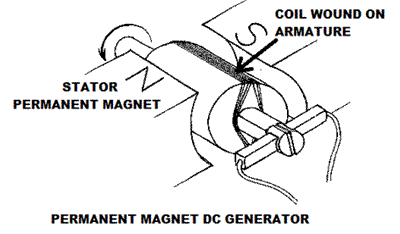When the flux in the magnetic circuit is established by the help of permanent magnets then it is known as Permanent magnet DC generator.
It consists of an armature and one or several permanent magnets situated around the armature. This type of DC generators generates very low power. So, they are rarely found in industrial applications. They are normally used in small applications like dynamos in motor cycles.

### Separately Excited DC Generator

These are the generators whose field magnets are energized by some external dc source such as battery .
A circuit diagram of separately excited DC generator is shown in figure. Ia = Armature current IL = Load current V = Terminal voltage Eg = Generated emf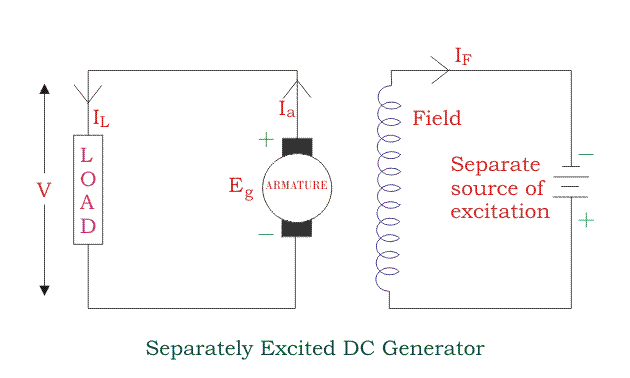Voltage drop in the armature = Ia × Ra(R/sub>a is the armature resistance) Let, Ia = IL = I (say) Then, voltage across the load, V = IRa Power generated, Pg = Eg×I Power delivered to the external load, PL = V×I.

### Self-excited DC Generators

These are the generators whose field magnets are energized by the current supplied by themselves. In these type of machines field coils are internally connected with the armature. Due to residual magnetism some flux is always present in the poles. When the armature is rotated some emf is induced. Hence some induced current is produced. This small current flows through the field coil as well as the load and thereby strengthening the pole flux. As the pole flux strengthened, it will produce more armature emf, which cause further increase of current through the field. This increased field current further raises armature emf and this cumulative phenomenon continues until the excitation reaches to the rated value. According to the position of the field coils the self-excited DC generators may be classified as…
1. Series wound generators
2. Shunt wound generators
3. Compound wound generators

#### Series Wound Generator

In these type of generators, the field windings are connected in series with armature conductors as shown in figure below. So, whole current flows through the field coils as well as the load. As series field winding carries full load current it is designed with relatively few turns of thick wire. The electrical resistance of series field winding is therefore very low (nearly 0.5Ω ). Let, Rsc = Series winding resistance Isc = Current flowing through the series field Ra = Armature resistance Ia = Armature current IL = Load current V = Terminal voltage Eg= Generated emf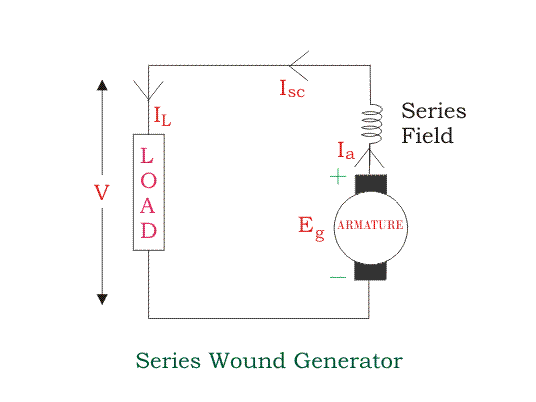Then, Ia = Isc = IL=I (say) Voltage across the load, V = Eg -I(Ia×Ra) Power generated, Pg = Eg×I Power delivered to the load, PL = V×I

#### Shunt Wound DC Generators

In these type of DC generators the field windings are connected in parallel with armature conductors as shown in figure below. In shunt wound generators the voltage in the field winding is same as the voltage across the terminal. Let, Rsh = Shunt winding resistance Ish = Current flowing through the shunt field Ra = Armature resistance Ia = Armature current IL = Load current V = Terminal voltage Eg = Generated emf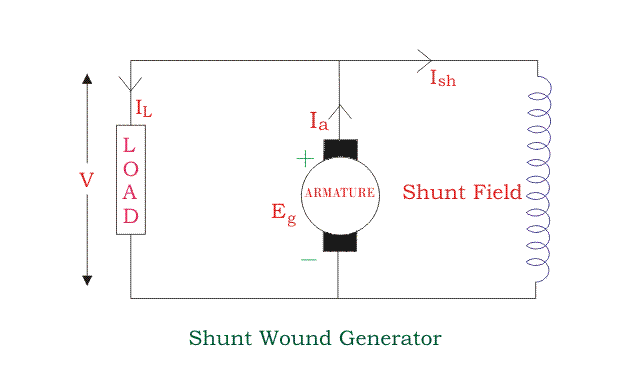Here armature current Ia is dividing in two parts, one is shunt field current Ish and another is load current IL. So, Ia=Ish + IL The effective power across the load will be maximum when IL will be maximum. So, it is required to keep shunt field current as small as possible. For this purpose the resistance of the shunt field winding generally kept high (100 Ω) and large no of turns are used for the desired emf. Shunt field current, Ish = V/RshVoltage across the load, V = Eg-Ia RaPower generated, Pg= Eg×Ia Power delivered to the load, PL = V×IL

#### Compound Wound DC Generator

In series wound generators, the output voltage is directly proportional with load current. In shunt wound generators, output voltage is inversely proportional with load current. A combination of these two types of generators can overcome the disadvantages of both. This combination of windings is called compound wound DC generator. Compound wound generators have both series field winding and shunt field winding. One winding is placed in series with the armature and the other is placed in parallel with the armature. This type of DC generators may be of two types- short shunt compound wound generator and long shunt compound wound generator.
##### Short Shunt Compound Wound DC Generator
The generators in which only shunt field winding is in parallel with the armature winding as shown in figure.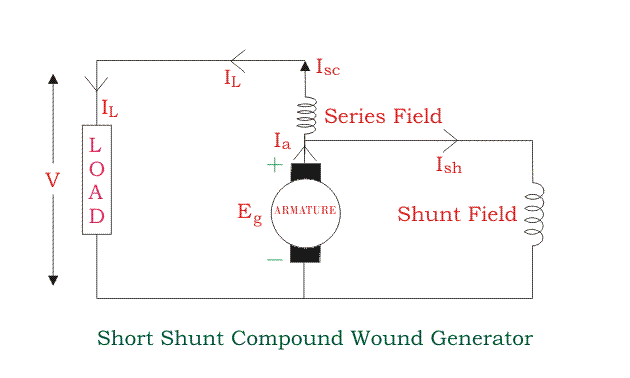Series field current, Isc = IL Shunt field current, Ish = (V+Isc Rsc)/RshArmature current, Ia = Ish + IL Voltage across the load, V = Eg - Ia Ra - Isc RscPower generated, Pg = Eg×Ia Power delivered to the load, PL=V×IL
##### Long Shunt Compound Wound DC Generator
The generators in which shunt field winding is in parallel with both series field and armature winding as shown in figure.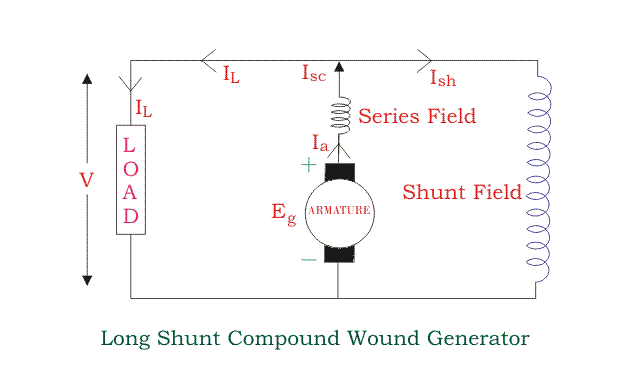Shunt field current, Ish=V/RshArmature current, Ia= series field current, Isc= IL+Ish Voltage across the load, V=Eg-Ia Ra-Isc Rsc=Eg-Ia (Ra+Rsc) [∴Ia=Ics] Power generated, Pg= Eg×IaPower delivered to the load, PL=V×ILIn a compound wound generator, the shunt field is stronger than the series field. When the series field assists the shunt field, generator is said to be commutatively compound wound. On the other hand if series field opposes the shunt field, the generator is said to be differentially compound wound.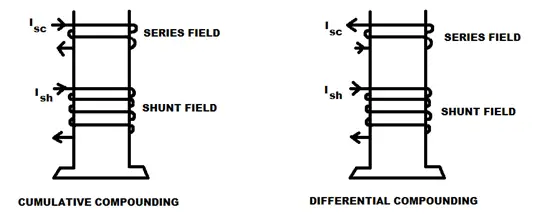vbn

# Characteristic of Shunt Wound DC Generator

In shunt wound DC generators the field windings are connected in parallel with armature conductors as shown in figure below. In these type of generators the armature current Iadivides in two parts. One part is the shunt field current Ish flows through shunt field winding and the other part is the load current IL goes through the external load.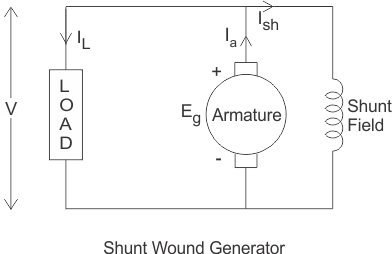Three most important characteristic of shunt wound dc generators are discussed below:

## Magnetic or Open Circuit Characteristic of Shunt Wound DC Generator

This curve is drawn between shunt field current(Ish) and the no load voltage (E0). For a given excitation current or field current, the emf generated at no load E0 varies in proportionally with the rotational speed of the armature. Here in the diagram the magnetic characteristic curve for various speeds are drawn.
Due to residual magnetism the curves start from a point A slightly up from the origin O. The upper portions of the curves are bend due to saturation. The external load resistance of the machine needs to be maintained greater than its critical value otherwise the machine will not excite or will stop running if it is already in motion. AB, AC and AD are the slops which give critical resistances at speeds N1, N2 and N3. Here, N1 > N2 > N3.

### Critical Load Resistance of Shunt Wound DC Generator

This is the minimum external load resistance which is required to excite the shunt wound generator.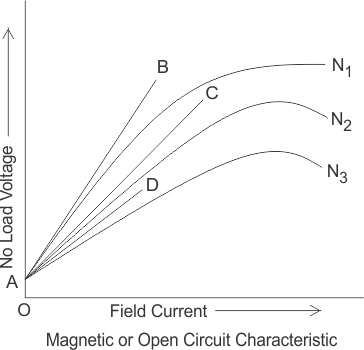## Internal Characteristic of Shunt Wound DC Generator

The internal characteristic curve represents the relation between the generated voltage Eg and the load current IL. When the generator is loaded then the generated voltage is decreased due to armature reaction. So, generated voltage will be lower than the emf generated at no load. Here in the figure below AD curve is showing the no load voltage curve and AB is the internal characteristic curve.

## External Characteristic of Shunt Wound DC Generator

AC curve is showing the external characteristic of the shunt wound DC generator. It is showing the variation of terminal voltage with the load current. Ohmic drop due to armature resistance gives lesser terminal voltage the generated voltage. That is why the curve lies below the internal characteristic curve.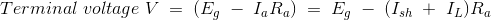The terminal voltage can always be maintained constant by adjusting the of the load terminal.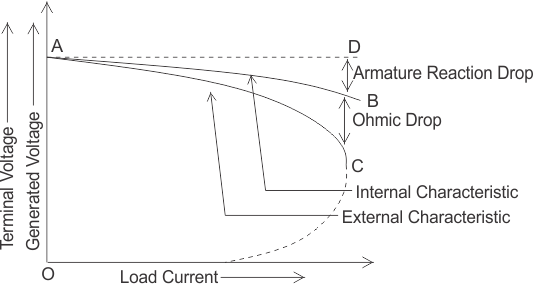When the load resistance of a shunt wound DC generator is decreased, then load current of the generator increased as shown in above figure. But the load current can be increased to a certain limit with (upto point C) the decrease of load resistance. Beyond this point, it shows a reversal in the characteristic. Any decrease of load resistance, results in current reduction and consequently, the external characteristic curve turns back as shown in the dotted line and ultimately the terminal voltage becomes zero. Though there is some voltage due to residual magnetism.
We know, Terminal voltage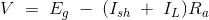Now, when IL increased, then terminal voltage decreased. After a certain limit, due to heavy load current and increased ohmic drop, the terminal voltage is reduced drastically. This drastic reduction of terminal voltage across the load, results the drop in the load current although at that time load is high or load resistance is low.
That is why the load resistance of the machine must be maintained properly. The point in which the machine gives maximum current output is called breakdown point (point C in the picture).